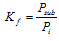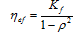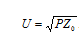# RF Power StandardStandard designation: RF power standard

Internal identifier: ECM 240-1/01-016

Year of approval: 2001

Department: Primary metrology of RF electrical quantities 1013, Regional branch Prague

Person in charge: Ing. Karel Dražil, Ing. František Hejsek (2001 – 2008)

Number of CMC rows: 7

Basic metrological parameters of the standard:

 Frequency range 1 MHz  -  40 GHz Power range (1  -  10) mW Calibration factor 0,6  -  1,2 Expanded uncertainty (k = 2) 0,0012  -  0,022

Measurement of "typical" electrical quantities (voltage, current) is very problematic in the area of radio-frequency (RF) and microwave frequencies. The RF power, however, is a quantity which is measured easily. The use of the RF power is advantageous for provision of measurement traceability, as one can use calorimetric methods which compare thermal effects of high frequency power and direct current, respectively. Measurement of RF power is of great importance for industry, for instance communications systems.

Basic elements of the national standard are the power meters with power sensors based on the principle of conversion of electrical to thermal energy. The metrological traceability is ensured by means of calorimetric methods. The laboratory does not operate any calorimeter due to economical reasons, the power sensors are periodically calibrated in other national metrology institutes (frequency dependence of the effective efficiency and calibration factor, respectively). The particular sensors are a N-type calorimetric power sensor, N-type thermistor power sensors, R220 and R320 waveguide thermistor power sensors and a 3.5mm thermoelectric power sensor.

Calibration factor Kf  of a power sensor is defined aswhere

 Psub. ...........… substitution power, which causes equivalent power meter reading, as the RF power, Pi .......…..... power of the wave incident to the power sensor.

For power sensors which determine the national standard traceability, the substitution power Psub is realized by means of a direct current with traceability to standards of "classical" electrical quantities. Thermistor power sensors are calibrated in other national metrology institutes operating a microcalorimeter, which is a primary standard ensuring the power sensor effective efficiency hef. The effective efficiency and calibration factor Kf  are related as followswhere r is the magnitude of the power sensor reflection coefficient.

Apart from power sensors, the national standard of RF power consists of voltmeters, signal generators, leveling three-ports (resistive power splitters and directional couplers), attenuators and leveling power meters. The measurement is carried out automatically with a control program. For calibration of lower-grade standards, a method of direct comparison of the standard and calibrated power sensor with the use of a power splitter or a directional coupler is used. The RF power and calibration factor (dimensionless) are the basic measured quantities. An attenuation is derived from a measurement of power ratio. In the area of high frequency measurements, also a RF voltage and RF current, respectively, are derived from the RF power. A voltage U in an impedance matched transmission line with the characteristic impedance Z0 (usually 50 Ω) can be calculated from a power P with use of following relationThe reflection coefficient is measured as a part of the power sensor calibration (important for the measurement uncertainty evaluation). The reflection coefficient is measured using a vector network analyzer (VNA) and therefore systematic errors caused by the impedance mismatch can be corrected.

Tha national standard has successfully participated in a KCDB key comparison. A two-side comparison (DUNAMET D-8) has been performed, too.